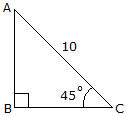# Engineering Mechanics - General Principles - Discussion

### Discussion :: General Principles - General Questions (Q.No.2)

2.Using the basic trigonomic functions, determine the length of side AB of the right triangle.

 [A]. h = 7.07 [B]. h = 10 [C]. h = 5 [D]. h = 14.14

Explanation:

No answer description available for this question.

 Rathod Vishal said: (Nov 23, 2010) sin45=AB/10 .707=AB/10 AB=7.07

 Nagaraju said: (Nov 24, 2010) Sin45 value = 1/1.414 = 0.707213579

 Prem said: (Dec 16, 2010) Sin 45 = AB/AC so, AB = AC x Sin45 AB = 10 x Sin 45 AB = 7.07

 Raji said: (Jan 4, 2011) From the given diagram, let AB=BC=x and AC=d x^2 + x^2 = d^2 2(x^2) =d^2 on Substituting the value of d=10 2(x^2) = 100 x^2 = 50 = 2x25 x = 5 x [(2)^(1/2)] = 5 x 1.141 = 7.07

 Yramakrishna said: (Feb 21, 2011) sin45=AB/10 AB=7.07

 Ramji said: (Mar 16, 2011) sin45 = 0.70 0.70*10 = 7.07

 Ashwini said: (Mar 16, 2011) Let base and height= x (becoz angle is 45) So x2+X2= 2X2=100 HENCE X=7.07

 Madan said: (Apr 22, 2011) Sin45=ab/ac where ac=10 then ab=sin45*ac ab=.707*10 ab=7.07

 Jai Praksh Singh said: (Jun 3, 2011) Sin 45 = AB/AC =AB/10 AB = .0707X10 = 7.07 AB=7.07

 G.Kavipandi said: (Jul 26, 2011) AB=sin45*10 =7.07

 G.Kavipandi said: (Jul 26, 2011) AB=sin theta * x .it direct formula to find distance in em

 Ashok said: (Aug 22, 2011) AB=BC*AC AB=SIN45*10 =0.707*10 AB=7.07

 Manju G said: (Sep 13, 2011) Its very to find out, Sin 45 = opp/ hy opp = sin 45 x hy = 1/sq.root of 2 x 10 opp = 7.07

 Ajit Banne Bec Bagalkot said: (Oct 31, 2011) AB = ACsin45 AB = 10*0.707 AB = 7.07

 Saharsh said: (Jan 17, 2012) Perpendicular AB sin(45)= ---------------- = ------ hypotenuse 10 So, AB = sin(45)x 10 = 0.707x10 = 7.07 Ans.

 Bepo said: (Jan 23, 2012) sin 45=AB/AC 1/2^2=AB/10 10* 1/1.4142=AB/10 10* 0.707=AB 7.07=AB

 Prakash said: (Jan 28, 2012) sin theta=opp. side/hyp. sin45=AB/10 .707=AB/10 AB=.707*10 AB=7.07

 Daulu Reve said: (Feb 10, 2012) sin45=AB/AC sin45=AB/10 AB=sin45*10 AB=7.07

 Nikhil said: (Feb 15, 2012) @Vishal method is easy.

 Raja said: (May 23, 2012) @Manju's method is easy.

 Marghoobul Islam said: (Jun 15, 2012) H/B=SIN 45*. =>AB/AC= SIN 45*. =>AB/10=0.707106781. =>AB=0.707106781*10. =>AB=7.07.

 Sudhakar said: (Aug 30, 2012) In Given Triangle by using trignomtric relations sin (theta)= AB/AC=AB/10 we know that sin45=0.707 0.707=AB/10 =>AB=0.707*10

 Kabaru said: (Jan 19, 2014) SOH-CAH-TOA. SIN(THETA) = OPPOSITE/HYPOTENUSE. COS(THETA) = ADJACENT/HYPOTENUSE. TAN(THETA) = OPPOSITE/ADJACENT. THEREFORE AB = CAH. AB = COS (THETA) = adj/hyp. AB = COS 45 = ADJ/10. AB = 10. COS45. And, COS45 = 0.707, AB = 10 . 0.707 AB = 7.07.

 Ashutosh said: (Feb 2, 2014) sin(45) = AB/10. AB = 10sin(45). AB = 10^0.707. AB = 7.07.

 Mani said: (Mar 10, 2014) Sin 45 = AB/10. 0.707*10 = AB. AB = 7.07.

 Anisha said: (May 18, 2014) How to calculate hypotenuse of a right triangle?

 Darshan Deore said: (Oct 5, 2014) Sin45 = AB/10. 0 = AB/10. AB = 7.07.

 Sunil Mlaviya said: (Oct 22, 2014) sin 45 = ab/10. (sin 45 = 0.07) ab = .07x10. ab = 7.07.

 Venkateshwaran said: (Apr 6, 2015) Without calculator how will you find out sin 45 is 0.707. Did you use any tricks?

 Nitika Arora said: (Sep 28, 2015) AB = Perpendicular; AC = hypotenuse. Now we know sin 45 = AB/AC. ->> 1/sqrt2 = AB/10. ->> 0.707 = AB/10 (1/sqrt2 = 0.707). ->> AB = 10*0.707. ->> AB = 7.07.

 Md.Aminurrahman said: (Jul 16, 2016) Sine45 degree = AB\10. AB = 7.07 => Answer.

 Sanket Kamble Pce said: (Feb 13, 2017) Sin 45 = AB/10. 0.707 * 10 = AB. AB = 7.07.

 Ravikumar said: (Jun 13, 2017) Sin θ =opposite side/hypotenuse sin(theera) = AB/ AC. AB=Ac sin45. = 10 sin45= 10 * 0.707. So, AB = 7.07.

 Janardhana said: (Jul 29, 2017) You are correct @Ashwini.